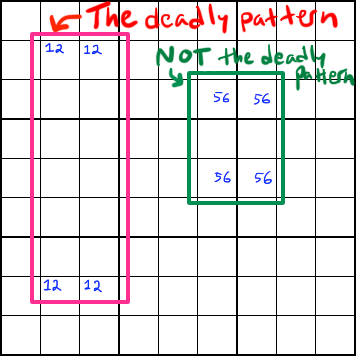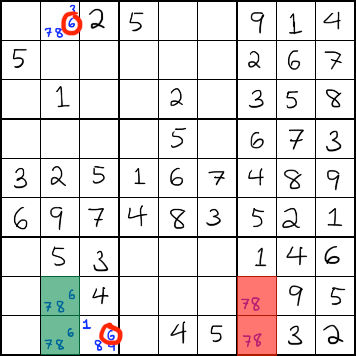# Unique Rectangle Strategy

The unique rectangle strategy can only be used on puzzles that have one possible solution. They take advantage of the fact that certain arrangements of candidates would lead to a puzzle with multiple solutions. Based on the rules below, you can eliminate candidates that would lead to multiple solutions.

The unique rectangle strategy focuses on a particular arrangement of candidates that is sometimes referred to as the "deadly pattern." In this pattern, there are four squares in a rectangular formation that each contain the same two candidates, along with other candidates in at least one square. In addition, the rectangle occupies exactly two boxes.The above image illustrates the idea of a unique rectangle. The one on the left is a unique rectangle. The candidates that form the rectangle occupy only two boxes. When solved, the 1s could be in the top left and bottom right and the 2s in the top right and bottom left, but you could also switch them around and do it the other way. It would still produce a completely valid solution because the numbers would be in the same two boxes, and there is no way that switching the numbers could produce a conflict. Since most sudoku puzzles are designed to have only one solution, you can't allow the candidates in your solution to form the deadly pattern, because it would lead to a puzzle with multiple solutions. This is the danger of the deadly pattern.

The green rectangle, though, is not a unique rectangle. Each corner is in a different box. The 5s could be in the top left and bottom right and the 6s in the top right and bottom left, or the other way around. However, only one of those configurations can be the correct one. That's because switching the numbers would put different numbers into different boxes. One arrangement will produce a conflict and the other will not. Therefore, this arrangement of candidates is safe, and NOT a deadly pattern.

### One corner has non-rectangle candidates

In the simplest type of unique rectangle, one of the rectangle's corners contains candidates that aren't part of the unique rectangle.

(Play this Example)In this example, the numbers 2 and 3 could potentially form a deadly pattern. However, the bottom-right corner (shown with the red arrow) contains numbers that aren't part of the deadly pattern. Because of this, you can remove the 2 and 3 from that cell.

Why do you remove both and not just one? Because if that cell is a 2, it would force the others to form the deadly pattern. If that cell is a 3, it would force the others to form the deadly pattern. Therefore, neither deadly pattern number can be correct for that cell.

### Two cells contain one non-deadly number and are in the same box

In the following examples, the unique rectangle cells that contain more than two numbers are referred to as the roof and the cells that only contain the deadly numbers are referred to as the floor.

(Play this Example)In this type of elimination, the roof cells (highlighted in green) are in the same box, and they both contain the same non-deadly candidate (in this case 6). In order to thwart the deadly pattern, one of the roof cells MUST be 6. We don't know which one. However, we can look for that same candidate in cells that both of the roof cells can see. Since one of the roof cells must be that non-deadly candidate, neither of the circled red 6s can be correct, so we can eliminate them.

### Two cells contain one non-deadly number but are in different boxes

This type is almost exactly the same as the previous type, except that the rectangle is rotated such that the roof is split between two different boxes, and so is the floor. The roof shares one non-deadly candidate, and you can remove that candidate from any cells that both roof cells can see.

### Roof contains a pair

If the roof cells contain two non-deadly numbers, or if one of them contains two and the other contains one of those two, then you can eliminate those two numbers from any cells that both roof cells can see.

### One of the deadly numbers in the roof forms a conjugate pair, and roof is in same box

If the roof cells are both in the same box, and if one of the deadly numbers ONLY occurs in the roof and nowhere else in the box, then in order to prevent the deadly pattern, you have to remove the other deadly pattern number from the roof.

### One of the deadly numbers in the roof forms a conjugate pair, and roof is in two different boxes

Just as with the previous type, if one of the deadly pattern numbers forms a conjugate pair in the roof, you can delete the other deadly number from the roof.